Next: Example 10.6: Impedance matching Up: Inductance Previous: Example 10.4: The RC

Example 10.5: The step-up transformer

Question: An electric power plant produces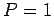GW of AC electric power at a peak voltage of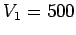V. If it is desired to transmit this power at a peak voltage ofkV, what is the appropriate turns-ratio of the step-up transformer? What peak current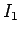would be sent over the transmission line if the peak voltage wereV? What peak current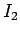would be sent over the transmission line if the peak voltage werekV? What is the ratio of the ohmic powers losses in the line in the two cases?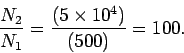Since the peak power is given by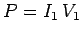, it follows that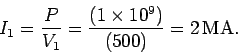Since the peak power remains unchanged after the signal passes through the transformer (assuming that there are no power losses in the transformer), we have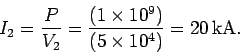The ratio of the power lost to ohmic heating in the two cases iswhereis the resistance of the transmission line. Note that the ohmic power loss is much greater at low peak voltage than at high peak voltage.Next: Example 10.6: Impedance matching Up: Inductance Previous: Example 10.4: The RC
Richard Fitzpatrick 2007-07-14